Next: Newton's second law of Up: Newtonian mechanics Previous: Newton's laws of motion

# Newton's first law of motion

Newton's first law of motion essentially states that a point object subject to zero net external force moves in a straight line with a constant speed (i.e., it does not accelerate). However, this is only true in special frames of reference called inertial frames. Indeed, we can think of Newton's first law as the definition of an inertial frame. Thus, an inertial frame of reference is one in which a point object subject to zero net external force moves in a straight line with constant speed.

Suppose that we have found an inertial frame of reference. Let us set up a Cartesian coordinate system in this frame. The motion of a point object can now be specified by giving its position vector,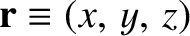, with respect to the origin of the coordinate system, as a function of time,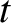. Consider a second frame of reference moving with some constant velocity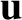with respect to the first frame. Without loss of generality, we can suppose that the Cartesian axes in the second frame are parallel to the corresponding axes in the first frame, that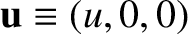, and, finally, that the origins of the two frames instantaneously coincide at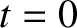. (See Figure 2.1.) Suppose that the position vector of our point object is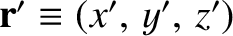in the second frame of reference. It is evident, from Figure 2.1, that at any given time,, the coordinates of the object in the two reference frames satisfy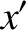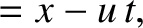(2.1)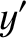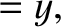(2.2) and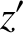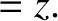(2.3)

This coordinate transformation was first discovered (implicitly) by Galileo Galilei (1564-1642), and is nowadays known as a Galilean transformation in his honor.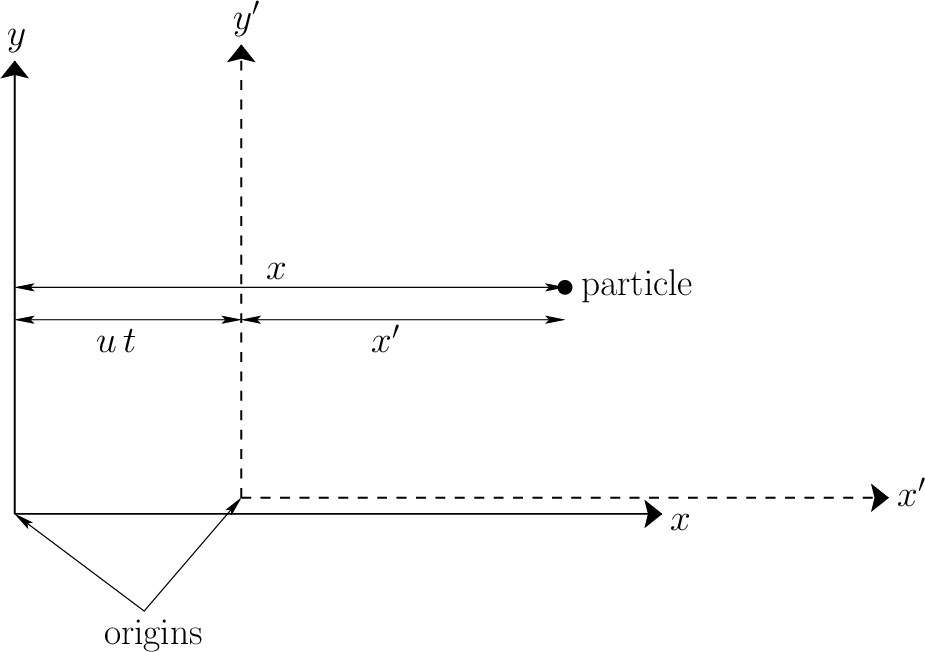By definition, the instantaneous velocity of the object in our first reference frame is given by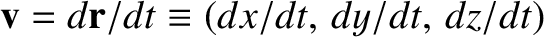, with an analogous expression for the velocity,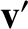, in the second frame. It follows, from differentiation of Equations (2.1)-(2.3) with respect to time, that the velocity components in the two frames satisfy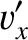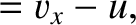(2.4)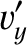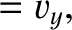(2.5) and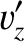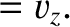(2.6)

These equations can be written more succinctly as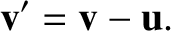(2.7)

Finally, by definition, the instantaneous acceleration of the object in our first reference frame is given by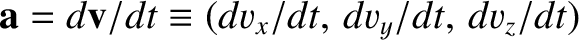, with an analogous expression for the acceleration,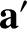, in the second frame. It follows, from differentiation of Equations (2.4)-(2.6) with respect to time, that the acceleration components in the two frames satisfy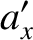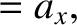(2.8)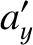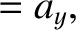(2.9) and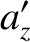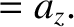(2.10)

These equations can be written more succinctly as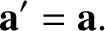(2.11)

According to Equations (2.7) and (2.11), if an object moves in a straight line with a constant speed in our original inertial frame (i.e., if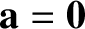) then it also moves in a (different) straight line with a (different) constant speed in the second frame of reference (i.e.,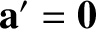). Hence, we conclude that the second frame of reference is also an inertial frame.

A simple extension of the preceding argument allows us to conclude that there exists an infinite number of different inertial frames moving with constant velocities with respect to one another. Newton thought that one of these inertial frames was special and defined an absolute standard of rest: that is, a static object in this frame was in a state of absolute rest. However, Einstein showed that this is not the case. In fact, there is no absolute standard of rest, which implies that all motion is relative--hence, the name relativity'' for Einstein's theory. Consequently, one inertial frame is just as good as another as far as Newtonian mechanics is concerned.

But what happens if the second frame of reference accelerates with respect to the first? In this case, it is not hard to see that Equation (2.11) generalizes to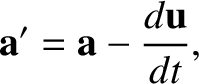(2.12)

where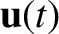is the instantaneous velocity of the second frame with respect to the first. According to this formula, if an object moves in a straight line with a constant speed in the first frame (i.e., if) then it does not move in a straight line with a constant speed in the second frame (i.e.,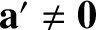). Hence, if the first frame is an inertial frame then the second is not.

A simple extension of the preceding argument allows us to conclude that any frame of reference that accelerates with respect to a given inertial frame is not itself an inertial frame.

For most practical purposes, when studying the motions of objects close to the Earth's surface, a reference frame that is fixed with respect to this surface is approximately inertial. However, if the trajectory of a projectile within such a frame is measured to high precision then it will be found to deviate slightly from the predictions of Newtonian mechanics. (See Chapter 6.) This deviation is due to the fact that the Earth is rotating about a north-south axis, and its surface is therefore accelerating towards this axis. When studying the orbits of objects around the Earth, a reference frame whose origin is the center of the Earth (or, to be more exact, the center of mass of the Earth-Moon system), and whose coordinate axes are fixed with respect to distant stars, is approximately inertial. However, if such orbits are measured to extremely high precision then they will again be found to deviate very slightly from the predictions of Newtonian mechanics. In this case, the deviation is due to the Earth's orbital rotation about the Sun. When studying the orbits of the planets in the solar system, a reference frame whose origin is the center of the Sun (or, to be more exact, the center of mass of the solar system), and whose coordinate axes are fixed with respect to distant stars, is approximately inertial. In this case, any deviations of the orbits from the predictions of Newtonian mechanics due to the orbital rotation of the Sun about the galactic center are far too small to be measurable. It should be noted that it is impossible to identify an absolute inertial frame--the best approximation to such a frame would be one in which the cosmic microwave background appears to be (approximately) isotropic. However, for a given dynamical problem, it is always possible to identify an approximate inertial frame. Furthermore, any deviations of such a frame from a true inertial frame can be incorporated into the framework of Newtonian mechanics via the introduction of so-called fictitious forces. (See Chapter 6.)Next: Newton's second law of Up: Newtonian mechanics Previous: Newton's laws of motion
Richard Fitzpatrick 2016-03-31